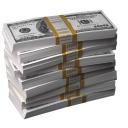# Finance 101 by TaiichiIn Chapter Six of Taiichi Ohno’s Workplace Management we read about the Profit formulas.  I must confess I thought I completely understood this concept when in fact I was only partly correct.  Let’s explore it tonight.  When we think about how a company can turn a profit there are three ways we can express it.

1. Price – Cost = Profit
2. Profit = Price – Cost
3. Price = Cost + Profit

For the math gurus out there you may be scratching your head as algebraically these formulas are the same.  But Mr. Ohno begs to differ.

Formula 3

Let’s start with the third formula (Price = Cost + Profit).  Mr. Ohno explains that this may be likened to the government approach to setting a price.  If, for example, a profit of \$100 is wanted and the cost to produce the product is \$200 the price is \$300.

Formula 2

This is the trickiest formula (Profit = Price – Cost) and is where I was a bit mistaken myself.  Ohno likens this formula to a company that produces luxury goods since they cannot reduce costs.  I am reminded of a recent article I read about Motorola who is targeting the rich with their newest Razr like cell phone. I wish they would wake up and just get their costs down by implementing some Lean with all that Six Sigma… but I digress.

Formula 1

Lastly we come to the Lean formula (Price – Cost = Profit).  This formula is arranged in such a way as to say that costs exist to be reduced, not to be calculated.  The thinking here is that the market sets the price and the only sure way we can increase profits is by reducing costs.